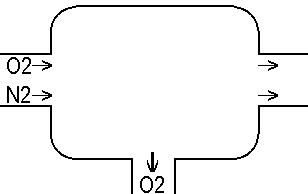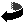# Karl´s Rebreather Page

## SCR-Calculations

Let's start it classic by beginning with the open Diving Bell.
In this system a flow of fresh gas is added to supply the diver with oxygen and wash out the carbon dioxide the diver exhales. We just have to make a balance to calculate what goes on.
Lets say the supply Air-flow is 100bar*l/min, the diver consumes 1bar*l/min Oxygen and so exhales 1barl*l/min CO2 . So the Loop dumps 21%*100bar*l/min - 1bar*l/min = 20bar*l/min Oxygen, 1bar*l/min CO2 and 79%*100bar*l/min N2.
This means the O2-Fraction inside the Bell is (21%*100bar*l/min - 1bar*l/min) / (100bar*l/min) = 20%,
the CO2-Fraction = (1bar*l/min) / (100bar*l/min) = 1% .
If the diver works in 40m depth it means 5bar*1%CO2 = 0.05bar CO2-partial-pressure in his bell.A Semiclosed-Rebreather (SCR) is quite similar, but the CO2 is scrubbed, not washed out, so it does not appear in the balance. On a Active Flow-SCR, like the Dräger Atlantis/Dolfin, the %O2 is changing as function of the added mass-flow and your workload, so if you don't use a ppO2-measuring deco-computer you have to calculate with worst cases: you have to use the highest ppN2 for deco-calculations (means lowest ppO2) and the highest ppO2 for the O-tox.
Ok, first of all we need the constant flow, but that's just using a sonic-speed-nozzle. As long as the supply-pressure is at least 1.9 * the ambient-pressure that nozzle shape delivers a flow that depends on the (absolute) nozzle-supply pressure, but not on the ambient-pressure. This means if our nozzle-supply pressure is too low it restricts the usage-depth, and if the tanks are on the way to bottom out we have to quit before this forces the IP to drop. Hypoxia comes treading softly, so be careful, "Keep watching those Displays" and do all the checks at least as frequent as you learned.
Now back to the Oxygen-Fracture in the loop:
FiO2 = the Inspiratorive Fraction that is O2
VvO2 = the O2 your Body needs (from 0,5bar*l/min to 2,5bar*l/min)
Vs = the gas [bar*l/min] your active-flow delivers
FsO2 = the O2 fraction of the gas in your tank (NOAA2=Nitrox-36 means FsO2=0,36)
The Oxygen-Fraction in the loop is the Ratio of the total Oxygen-Flow (Added Oxygen - Consumed Oxygen) to the total Gas-Flow (Added Gas - Consumed Oxygen).
FiO2=((Vs*FsO2)-VvO2)/(Vs-VvO2)
Now we can calculate the EAD:
EAD[m]=(Depth[m]+10m)((1-FiO2)/0,79)-10m
EAD[m]=(Depth[m]+10m)((1-(((Vs*FsO2)-1,5bar*l/min)/(Vs-1,5bar*l/min)))/0,79)-10m

Passive Flow-SCR's :
Some older Passive Flow-SCR's don't have a Passive Variable Ratio and always add a fixed Ratio (20 to 25%) of the bar*l you breath, which means the deeper you are the more gas they waste. (Let's call this Passive-Mode 1.)
K = Ventilation Coefficent = The Ratio of the respiratory minute volume Vv [liter] to the Oxygen consumed by the body VvO2 [bar*liter] = Vv/VvO2
r is the dump-Ratio, for example 1/4.
K is here assumed to lie in the range of 15 to 30, the average human has K=25.
Let's assume that the gas is scubbered after it has passed the bellows-system.
The added Gas is now: Vs = VvO2*K*P*r
FiO2=(Vs*FsO2-VvO2)/(Vs-VvO2)
FiO2=((VvO2*K*P*r)*FsO2-VvO2)/((VvO2*K*P*r)-VvO2)
FiO2=VvO2*(K*P*r*FsO2-1)/VvO2*(K*P*r-1)
FiO2=(K*P*r*FsO2-1)/(K*P*r-1)

or more correctly, because of the consumed oxygen also causes an addition of fresh gas:
Vs = VvO2*((1-r)+K*P*r) were (1-r)*VvO2 is the CO2 that reaches the scrubber
so you get, using
FiO2= (Vs*FsO2-VvO2) / (Vs-VvO2)
FiO2= ((VvO2*((1-r)+K*P*r))*FsO2-VvO2) / ((VvO2*((1-r)+K*P*r))-VvO2)
FiO2= ((VvO2*(((1-r)+K*P*r))*FsO2-1)) / ((VvO2*((1-r)+K*P*r-1))
FiO2= ((VvO2*(((1-r)+K*P*r))*FsO2-1)) / ((VvO2*(K*P*r-r))
FiO2= ((VvO2*(((1-r)+K*P*r))*FsO2-1)) / (VvO2*r*(K*P-1))

Modern Passive Flow-SCR's are keyed to the breathing and the depth, using a special mechanic.
Let's say we have a Passive Variable Ratio GAS Addition Semi-Closed Circuit Rebreathers that is adjusted so, that it always delivers 1/4 the fresh bar*l than the volume (litre) you breath. (Let's call this Passive-Mode 2.)
On the surface (1bar) it adds 5 bar*l/min while you breath 20bar*l/min,
in 30m (4bar) it still adds 5 bar*l/min while you still breath a volume of 20l/min although this means now 80bar*l/min. So you have the nice situation that the addition-flow is keyed to the O2 your body needs.

SD= r' =1/4 (in this example) = the ratio you dump (or scrub out) at the surface
SD= the ratio between breathed volume [l/min] * 1bar and the added gas [bar*l/min],
so it keeps the formula easy by including the scrubbed out CO2.
Vs=K*VvO2*SD (K=25, the average diver breaths with 0.04bar ppCO2-exhalation)
Now let's garb the normal SCR-Formula:
FiO2=((Vs*FsO2)-VvO2)/(Vs-VvO2)
insert the PVR-BASC and then reduce step by step to see what happens.
FiO2=(((K*VvO2*SD)*FsO2)-VvO2)/( K*VvO2*SD)-VvO2)
FiO2=((FsO2*(K*VvO2*SD))-VvO2)/((K*SD-1)*VvO2)
FiO2=(VvO2*((FsO2*(K*SD))-1)) / (VvO2*(K*SD-1))
FiO2=((FsO2*(K*SD))-1) / (K*SD-1)
Ok, now let's call K*SD=MV [=6.25 in our example]
FiO2=(FsO2*MV-1)/(MV-1)

For the average diver this 1/4 Surface-Dump-Ratio results in:

 FsO2 0.1 0.16 0.21 0.32 0.4 0.5 0.6 0.8 1 FiO2 deadly deadly 0.06 0.19 0.28 0.4 0.52 0.76 1

For a CO2-Retainer who breaths at ppCO2=0.08bar it results in:

 FsO2 0.1 0.16 0.21 0.32 0.4 0.5 0.6 0.8 1 FiO2 deadly deadly deadly deadly 0.11 0.26 0.41 0.7 1

For the average diver a 50% Surface-Dump-Ratio results in:

 FsO2 0.1 0.16 0.21 0.32 0.4 0.5 0.6 0.8 1 FiO2 0.021 caution! 0.086 0.14 0.26 0.34 0.45 0.56 0.78 1

For a CO2-Retainer who breaths at ppCO2=0.08bar it results in:

 FsO2 0.1 0.16 0.21 0.32 0.4 0.5 0.6 0.8 1 FiO2 deadly deadly 0.06 0.19 0.28 0.4 0.52 0.76 1

When you mix both passive modes, like it's possible with the Halcyon-Rebreather (PVR-BASC), (let's call this Passive-Mode 3) you have both, a mode that does not waste significant more gas then you are deeper but adds sufficent gas when you are deep and so have to dive deep-dive mixes. [ Sorry, i don't have a ratio-table of a Mode-3-Engine yet, so i can not offer you a fiO2(fsO2,depth)-Table for such an engine at the moment.]

My opinion is:
"For your own safety never dive any SCR without an electronic
that measures the ppO2 of the Gas that will be inhaled."

because that few bucks may save your live in case of a bug,
like grabbing the wrong tank or a silent increasing problem with the addition-nozzle or -ratio.Rebreather-Index

http://Rebreather.de/rebreather/scr.htm © Karl Kramer, 09.10.1998